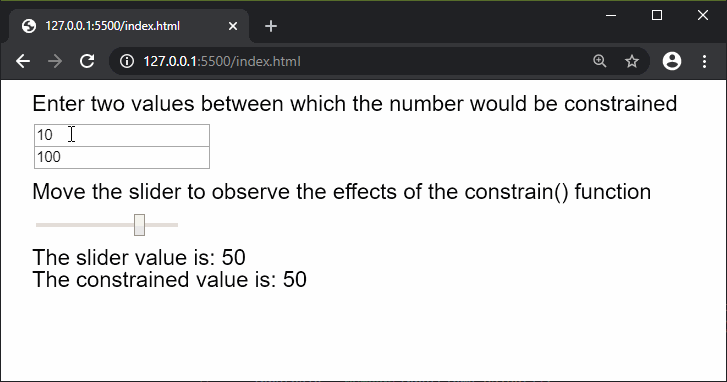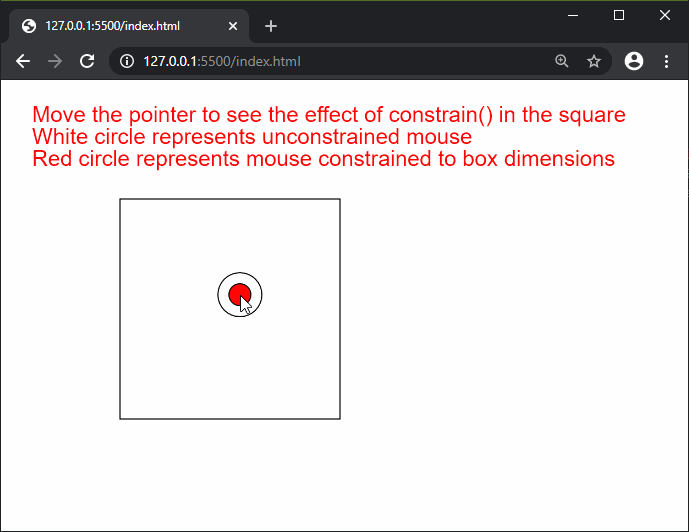# p5.js | constrain() Function

The constrain() function in p5.js is used to constrain a number between a given minimum and maximum limit.

Syntax:

`constrain( n, low, high )`

Parameters: This function accept three parameters as mentioned above and described below:

• n: It is a number which denotes the value that has to be constrained.
• low: It is a number which denotes the minimum limit to which the number is constrained.
• high: It is a number which denotes the maximum limit to which the number is constrained.

Return Value: It returns a number with the constrained value.

The example below illustrates the constrain() function in p5.js:

Example 1:

 `function` `setup() { ` `  ``createCanvas(650, 200); ` `  ``textSize(20); ` `  `  `  ``inputElemA = createInput(10); ` `  ``inputElemA.position(30, 40); ` `  `  `  ``inputElemB = createInput(100); ` `  ``inputElemB.position(30, 60); ` `  `  `  ``sliderElem = createSlider(-100, 100, 50, 1); ` `  ``sliderElem.position(30, 120); ` `} ` `  `  `function` `draw() { ` `  ``clear(); ` `  ``text(``"Enter two values between which the "` `    ``+ ``"number would be constrained"``, 20, 20); ` `  ``text(``"Move the slider to observe the effects"` `    ``+ ``" of the constrain() function"``, 20, 100); ` `  `  `  ``// Convert the string value to a number value ` `  ``inputValA = Number(inputElemA.value()); ` `  ``inputValB = Number(inputElemB.value()); ` `  ``sliderVal = sliderElem.value(); ` `  `  `  ``text(``"The slider value is: "` `+ sliderVal, 20, 160); ` `  `  `  ``// Display the constrained value ` `  ``text(``"The constrained value is: "` `        ``+ constrain(sliderVal, inputValA, ` `        ``inputValB), 20, 180); ` `} `

Output:Example 2:

 `function` `setup() { ` `  ``createCanvas(600, 350); ` `  ``textSize(20); ` `  `  `} ` `  `  `function` `draw() { ` `  ``clear(); ` `  ``text(``"Move the pointer to see the effect "` `        ``+ ``"of constrain() in the square"``, 20, 30); ` `  ``text(``"White circle represents unconstrained"` `        ``+ ``" mouse"``, 20, 50); ` `  ``text(``"Red circle represents mouse constrained"` `        ``+ ``" to box dimensions"``, 20, 70); ` `  `  `  ``noFill(); ` `  ``square(100, 100, 200); ` `  `  `  ``circle(mouseX, mouseY, 40); ` `  `  `  ``// Constrain the mouse x and y position ` `  ``constrainedMouseX = constrain(mouseX, 100, 300); ` `  ``constrainedMouseY = constrain(mouseY, 100, 300); ` `  `  `  ``fill(``'red'``); ` `  ``circle(constrainedMouseX, constrainedMouseY, 20); ` `} `

Output:Online editor: https://editor.p5js.org/My Personal Notes arrow_drop_upCheck out this Author's contributed articles.

If you like GeeksforGeeks and would like to contribute, you can also write an article using contribute.geeksforgeeks.org or mail your article to contribute@geeksforgeeks.org. See your article appearing on the GeeksforGeeks main page and help other Geeks.

Please Improve this article if you find anything incorrect by clicking on the "Improve Article" button below.

Article Tags :

Be the First to upvote.

Please write to us at contribute@geeksforgeeks.org to report any issue with the above content.